Command Reference : Operator and Function Reference : Basic Mathematical Functions

Basic Mathematical Functions
The following functions perform basic mathematical operations. When applied to a series, they return a value for every observation in the current sample. When applied to a matrix object, they return a value for every element of the matrix object. The functions will return NA values for observations where the input values are NAs, or where the input values are not valid. For example, the square-root function @sqrt, will return NAs for all observations less than zero.
A few of the functions take an optional argument at the end of the options list ([,n]). Non-zero values refer to the number of decimal points to take account of when performing the operation. For example, the @round function with an optional parameter of 2 will round to the nearest 100. @round with an optional parameter of -2 will round to the nearest 0.01.
Note: @iff, @inv, and @recode do not work with matrix objects.

 Name Function Examples/Description @abs(x), abs(x) absolute value @abs(-3)=3. @bounds(x,y,z) boundary values returns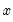ifis between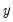and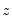and the boundary valuesandotherwise @ceiling(x[,n]) smallest integer not less than @ceiling(2.34)=3, @ceiling(4)=4. @exp(x), exp(x) exponential,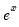@exp(1)=2.71813. @expm1(x) exponential,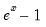For x near zero. @fact(x) factorial,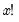@fact(3)=6, @fact(0)=1. @factlog(x) natural logarithm of the factorial,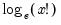@factlog(3)=1.79176, @factlog(0)=0. @floor(x[,n]) largest integer not greater than @floor(1.23)=1, @floor(-3.1)=-4. @iff(s,x,y) recode by condition returnsif condition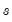is true; otherwise returns. Note this is the same as @recode. @inv(x) reciprocal,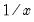inv(2)=0.5 (For series only; you should use @einv to obtain the element inverse of a matrix). @mod(x,y) floating point remainder returns the remainder of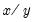with the same sign as. If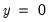the result is 0. @log(x), log(x) natural logarithm,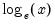@log(2)=0.693..., log(@exp(1))=1. @log1p(x)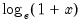Fornear zero. @log1mexp(x)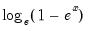For negativenear zero. @log10(x) base-10 logarithm,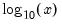@log10(100)=2. @logx(x,b) base-b logarithm,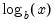@logx(256,2)=8. @nan(x,y) recode NAs in X to Y returnsif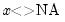, andif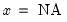. @pmax(x,y) pairwise max returns the pairwise max ofand(for more than pairs, use @rmax) @pmin(x,y) pairwise min returns the pairwise max ofand(for more than pairs, use @rmin) @pow(x,a) power,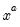@pow(2,3) = 8. @powm1(x,a)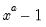Fornear one. @pow1pm1(x,a)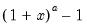Fornear zero. While similar to @powm1, this function supports a full double-precision “delta” from one. Among other things, this allows exponentiation of values extremely close to one that cannot be represented in double-precision, e.g.,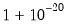. This function should only be used if the calculation ofnaturally falls near zero. If the calculation ofnaturally falls near one, @powm1 should be used instead. Supplying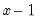to this function will produce less accurate results. @recode(s,x,y) recode by condition returnsif conditionis true; otherwise returns. @round(x[,n]) round to the nearest integer @round(-97.5)=-98, @round(3.5)=4. @sign(x) returns sign ofreturns -1, 0, 1 depending on the sign of the corresponding element of@sqrt(x), sqr(x) square root @sqrt(9)=3.